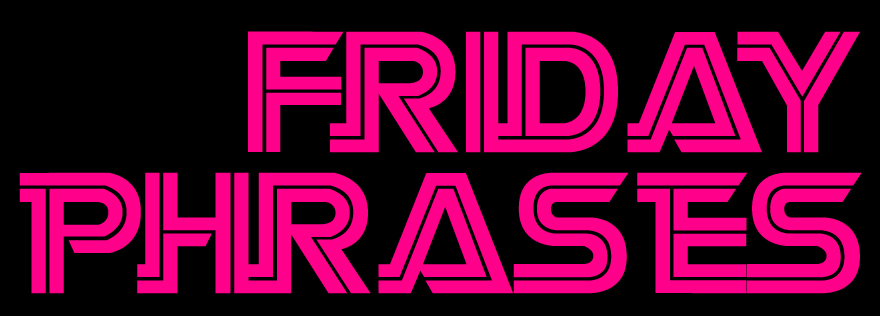June 14 2019

Friday Phrasesas we were= SWERP
as we were the= SWERPT
as we were it= SW*ERPT
as we were this= SWERPTS
as we were it is= SW*ERPTS
as we were to= SWERPTD
as we were to the= SW*ERPTD
one thing= WUNG
one thing is= WUNGS
one thing the= WUNGT
one thing it= W*UNGT
one thing this= WUNGTS
one thing it is= W*UNGTS
one thing to= WUNGTD
one thing to the= W*UNGTD